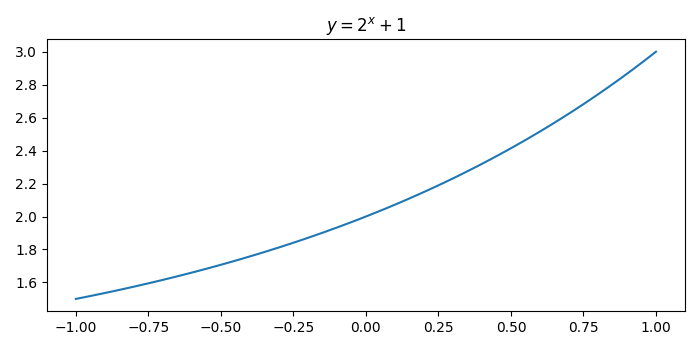# How to put a title for a curved line in Python Matplotlib?

To put a title for a curved line in Python Matplotlib, we can take the following steps −

## Steps

• Set the figure size and adjust the padding between and around the subplots.

• Create x and y data points such that the line would be a curve.

• Plot the x and y data points.

• Place a title for the curve plot using plt.title() method.

• To display the figure, use Show() method.

## Example

import matplotlib.pyplot as plt
import numpy as np

plt.rcParams["figure.figsize"] = [7.00, 3.50]
plt.rcParams["figure.autolayout"] = True

# Create x and y data points
x = np.linspace(-1, 1, 50)
y = 2**x + 1

# Plot the x and y data points
line, = plt.plot(x, y)

# Place a title on the plot
plt.title("$y=2^x+1$")

plt.show()


## Output

It will produce the following output −